Two-point charges +q and –q are held fixed at (–d, 0) and (d, 0) respectively of a (x, y) coordinate system. Then

(1) E at all points on the y-axis is along $\stackrel{^}{i}$

(2) The electric field $\stackrel{\to }{E}$ at all points on the x-axis has the same direction

(3) Dipole moment is 2qd directed along $\stackrel{^}{i}$

(4) Work has to be done in bringing a test charge from infinity to the origin

Concept Questions :-

Electric dipole
High Yielding Test Series + Question Bank - NEET 2020

Difficulty Level:

A point charge of 40 stat coulomb is placed 2 cm in front of an earthed metallic plane plate of large size. Then the force of attraction on the point charge is

(1) 100 dynes

(2) 160 dynes

(3) 1600 dynes

(4) 400 dynes

Concept Questions :-

Coulomb's law
High Yielding Test Series + Question Bank - NEET 2020

Difficulty Level:

Four charges equal to – Q are placed at the four corners of a square and a charge q is at its centre. If the system is in equilibrium the value of q is

1. $-\frac{Q}{4}\left(1+2\sqrt{2}\right)$

2. $\frac{Q}{4}\left(1+2\sqrt{2}\right)$

3. $-\frac{Q}{2}\left(1+2\sqrt{2}\right)$

4. $\frac{Q}{2}\left(1+2\sqrt{2}\right)$

Concept Questions :-

Coulomb's law
High Yielding Test Series + Question Bank - NEET 2020

Difficulty Level:

Which of the following graphs shows the variation of electric field E due to a hollow spherical conductor of radius R as a function of distance from the centre of the sphere

(1)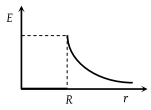(2)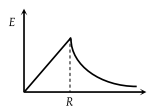(3)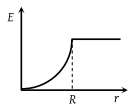(4)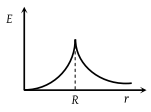Concept Questions :-

Electric field
High Yielding Test Series + Question Bank - NEET 2020

Difficulty Level:

The electric field due to a uniformly charged sphere of radius R as a function of the distance from its centre is represented graphically by

(1)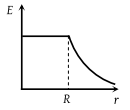(2)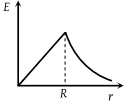(3)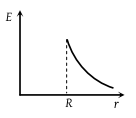(4)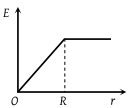Concept Questions :-

Electric field
High Yielding Test Series + Question Bank - NEET 2020

Difficulty Level:

Two concentric conducting thin spherical shells A and B having radii rA and rB (rB > rA) are charged to QA and $-{Q}_{B}\left(|{Q}_{B}|\text{\hspace{0.17em}}>\text{\hspace{0.17em}}|{Q}_{A}|\right)$. The electrical field at distance x from the common center is -

1.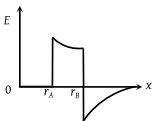2.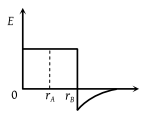3.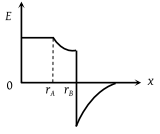4.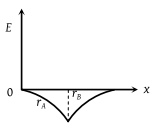Concept Questions :-

Electric field
High Yielding Test Series + Question Bank - NEET 2020

Difficulty Level:

Suppose the charge of a proton and an electron differ slightly. One of them is -e and the other is $\left(e+∆e\right)$. If the net of electrostatic force and gravitaional force between two hydrogen atoms placed at a distance d (much greater than atomic size) apart is zero,then $∆e$ is of the order [Given mass of hydrogen, ${m}_{h}$=1.67$×{10}^{-27}$ kg]

(a) ${10}^{-20}C$

(b)${10}^{-23}C$

(c) ${10}^{-37}C$

(d) ${10}^{-47}C$

Concept Questions :-

Coulomb's law
High Yielding Test Series + Question Bank - NEET 2020

Difficulty Level:

An electric dipole is place at an angle of ${30}^{\circ }$ with an electric field intensity 2$×{10}^{5}$ N/C. It experiences a torque equal to 4 Nm. The charge on the dipole, if the dipole length is 2 cm, is

(a) 8 mC              (b) 2 mC

(c) 5 mC              (d) 7 $\mu$

Concept Questions :-

Electric dipole
High Yielding Test Series + Question Bank - NEET 2020

Difficulty Level:

Two identical charged spheres suspended from a common point by two massless strings of lengths l are initially at a distance d(d < < l) apart because of their mutual repulsion. The charges begin to leak from both the spheres at a constant rate. As a result, the spheres approach each other with a velocity v. Then, v varies as a function of the distance x between the sphere, as

(a)

(b)

(c)

(d)

Concept Questions :-

Coulomb's law
High Yielding Test Series + Question Bank - NEET 2020

Difficulty Level:

The electric field in a certain region is acting radially outward and is given by E=Ar. A charge contained in a sphere of radius 'a' centered at the origin of the field will be given by

1. $4\pi {\in }_{o}A{a}^{2}$

2. $\pi {\in }_{o}A{a}^{2}$

3. $4\pi {\in }_{o}A{a}^{3}$

4. ${\in }_{o}A{a}^{2}$

Concept Questions :-

Gauss law